### IMO Shortlist 1988 problem 12

Kvaliteta:
Avg: 0,0
Težina:
Avg: 0,0
In a triangle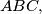$ABC,$ choose any points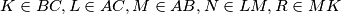$K \in BC, L \in AC, M \in AB, N \in LM, R \in MK$ and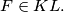$F \in KL.$ If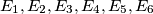$E_1, E_2, E_3, E_4, E_5, E_6$ and$E$ denote the areas of the triangles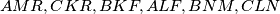$AMR, CKR, BKF, ALF, BNM, CLN$ and$ABC$ respectively, show that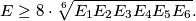Izvor: Međunarodna matematička olimpijada, shortlist 1988